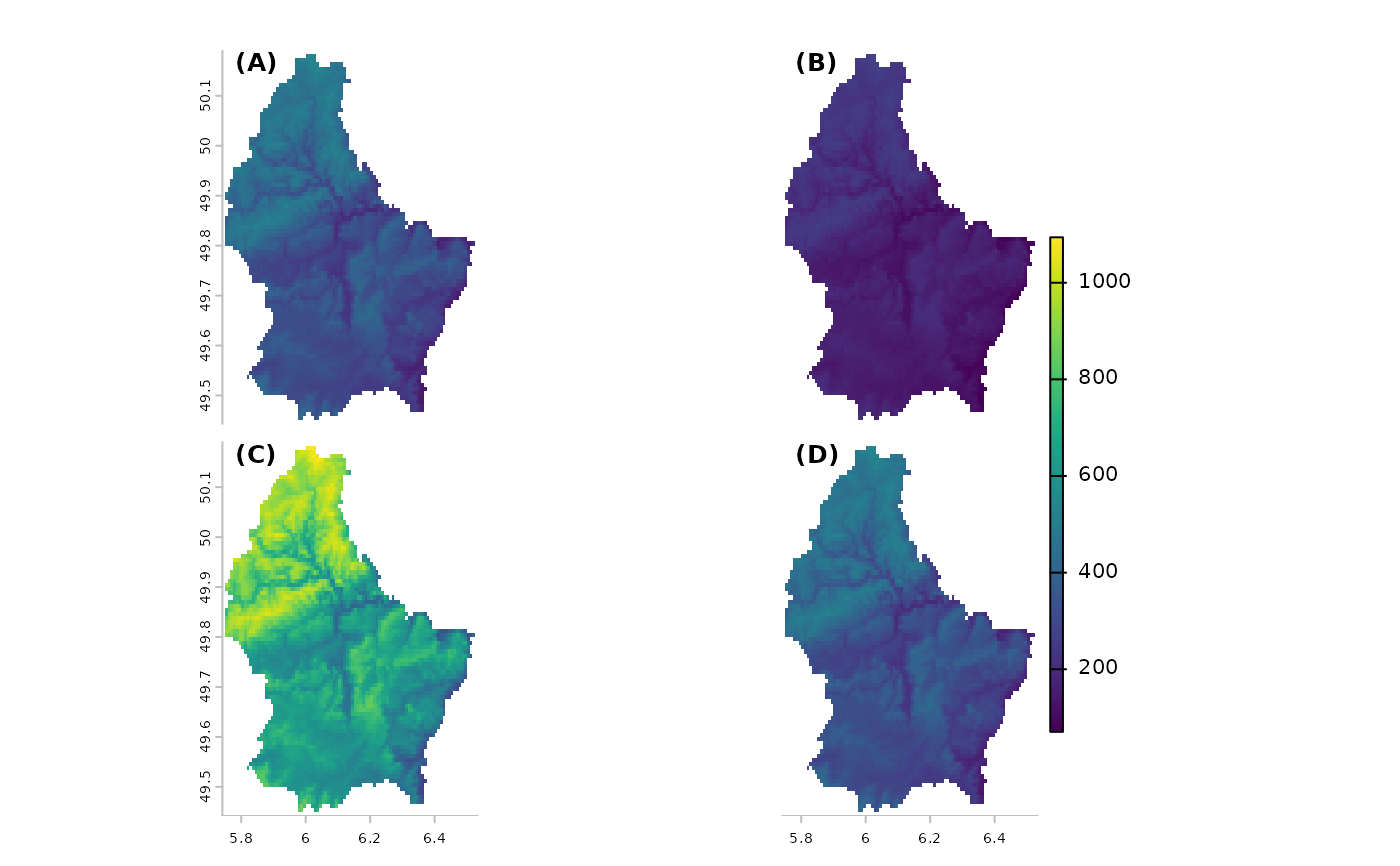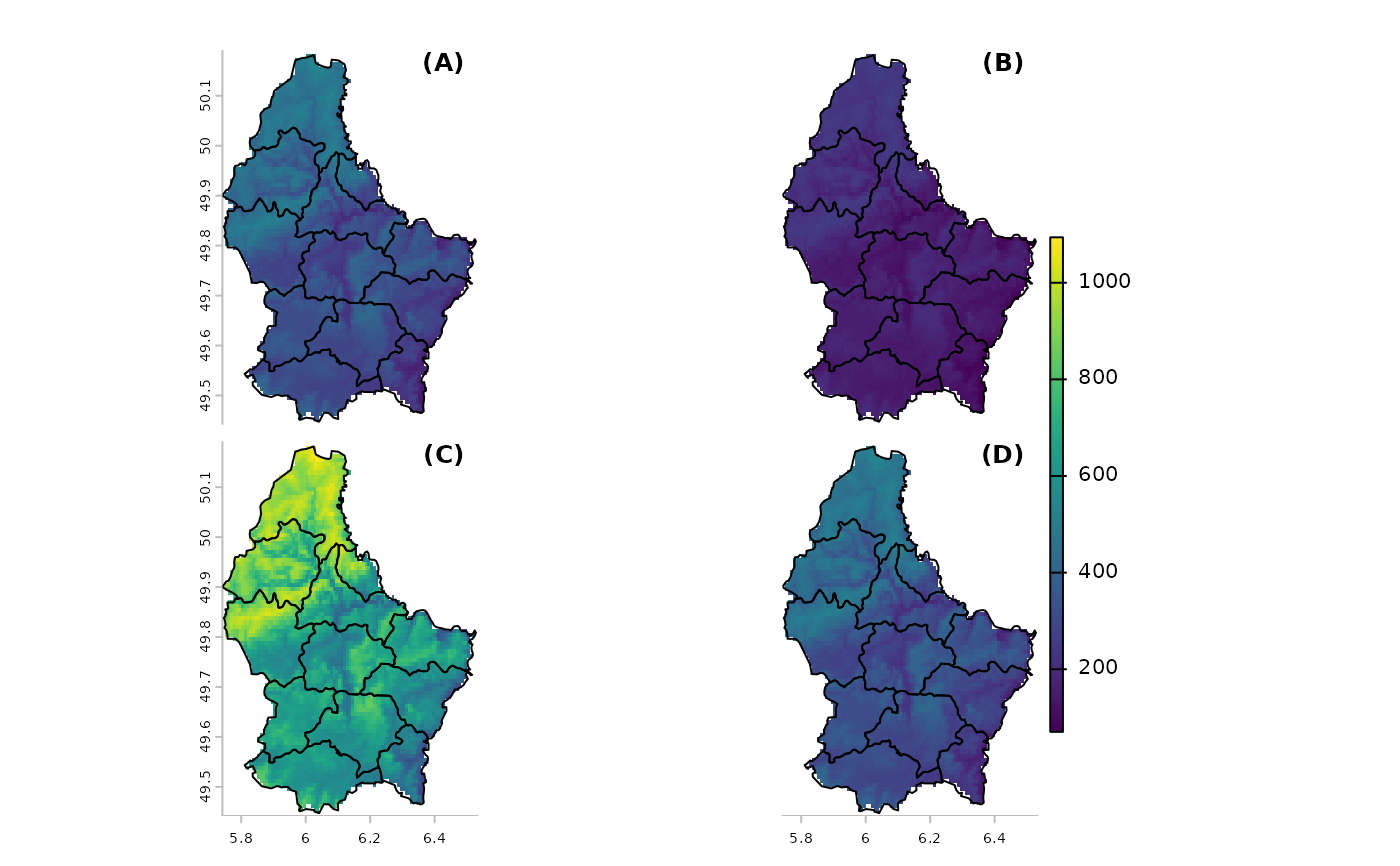Show multiple maps that share a single legend.

# S4 method for SpatRaster
panel(x, main, loc.main="topleft", nc, nr, maxnl=16,
maxcell=500000, box=FALSE, pax=list(), plg=list(), ...)

## Arguments

x

SpatRaster

main

character. Main plot titles (one for each layer to be plotted). You can use arguments cex.main, font.main, col.main to change the appearance

loc.main

numeric of character to set the location of the main title. Either two coordinates, or a character value such as "topleft")

nc

positive integer. Optional. The number of columns to divide the plotting device in (when plotting multiple layers)

nr

positive integer. Optional. The number of rows to divide the plotting device in (when plotting multiple layers)

maxnl

positive integer. Maximum number of layers to plot (for a multi-layer object)

maxcell

positive integer. Maximum number of cells to use for the plot

box

logical. Should a box be drawn around the map?

plg

see plot

pax

see plot

...

arguments passed to plot("SpatRaster", "numeric") and additional graphical arguments

plot and see rasterVis::levelplot and tidyterra::autoplot for more sophisticated panel plots.

## Examples

r <- rast(system.file("ex/elev.tif", package="terra"))
v <- vect(system.file("ex/lux.shp", package="terra"))
x <- c(r, r/2, r*2, r)
names(x) <- paste0("(", LETTERS[1:4], ")")
panel(x)panel(x, fun=\()lines(v), loc.main="topright")